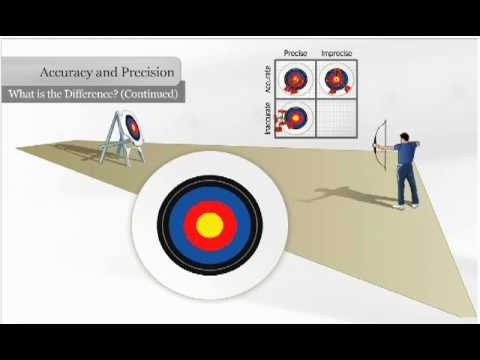### Main Difference

The main difference between accuracy and precision is that accuracy refers to the degree to which the measurement or calculation conforms to the exact value. Accuracy is exactness, validity and perfection. While precision is the attribute of the calculation to be consistently reproduced. Precisely, accuracy is very much close to exactness and correctness while precision lacks a block or two.

### Precision vs. Accuracy

Measurements are an important part of physics. Whenever a calculation is made, there is always a value obtained which can be correct or incorrect depending on the criteria set. These measurements can be termed many ways such as real, unreal, correct or incorrect when it comes to general explanation. But in a scientific way, there are two words which describe how real the calculation which has been done is. These terms are known as precision and accuracy. Both of them are usually considered to be the same and are used in that way, but in actual they are different from each other according to the way they are used and their exact meaning. This paragraph will explain the main differences between them for eliminating the confusion. Accuracy, in simple words, can be defined as something which is the measure of how close a value is to the standard which is set. Precision, on the other hand, is the closeness between different values which are taken over a period of time. The best example of these two can be an experiment conducted in a lab. For instance, if there are a bar and its thickness is measured. The answer comes out to be 5.3 cm. But when you check the actual value of the bar and its depth you see that the correct answer is 6.2 cm. It means the value is slightly inaccurate. Similarly, when three different outcomes for the thickness are obtained, let us say 6.3, 6.3, 6.2 in three repetitions the value is said to be precise. These terms are also used in real life, people call something accurate, especially a statement which is true in all sense and is based on a fact. Speaking linguistically, precise words can be the exact words which are spoken at the right time. These have wider use in sports too, in cricket if the bowler hits the wicket with the ball, it will be said that he is bowling accurately. On the other hand, if the bowler is bowling at the same length, again and again, the bowler will be bowling precisely. There are many other ways and examples to elaborate their difference but the above mentioned will be enough to create a better understanding. Further elaboration on these two types of scientific terms is given in the following paragraphs.

### Comparison Chart

 Basis Precision Accuracy Definition How close the values that have been taken are close to each other How close the answer is to the actual answer Number To check the precise measurement, the experiments have to be more than one. Accurate measurement can be one Statement True at all times True at some times Example The best example of accurate measurement can be a basketball game, in which the ball is thrown towards the basket if it goes in the basket the throw is accurate If the ball hits the same spot, again and again, the throws are called precise.

### What is Accuracy?

In a strict sense of view, accuracy shows how close a calculation is to the value that is given. Broadly stating, accuracy is very close to the target. The accuracy, for instance, of an experimental object is the value of how close the results match to the actual or known value given. Accuracy is also used as a statistical measure and it is the collection of true results rather than false ones. If the accuracy is of hundred percent then it concludes that calculated values are same as the given values.

### What is Precision?

On the other hand, precision is the number of true positives against the number of both, true and false positives. It actually refers to what is the degree to which something is strictly exact. Even if the measurements are far from the given value, precision indicates how reproducible they really are. And as for hitting the target, precisely hitting the given target means that all the arrows hit are spaced closely even if they are far from the main target.

### Key Differences

• Accuracy is correctness, while precision is close to exactness.
• Precision may denote the quality of an instrument, highly. While accuracy will not show the quality.
• There is a chance of further improvement in accuracy by taking repeated measurements and by doing repeated calculation. But, in the case of precision, it could not be improved by doing repeated calculations.
• Mathematically stating, accuracy is actually the degree of closeness to the given answer or value while precision is the degree of deviation from the original given result
• An accurate value can also be precise. But a precise value cannot be accurate.
• A value can be accurate occasionally, in a strict sense of view. But, a value can be precise many times.
• In accuracy, a single factor is needed. While in precision, multiple factors are needed.
• One cannot achieve accuracy without precision. But, precision could be achieved without accuracy.